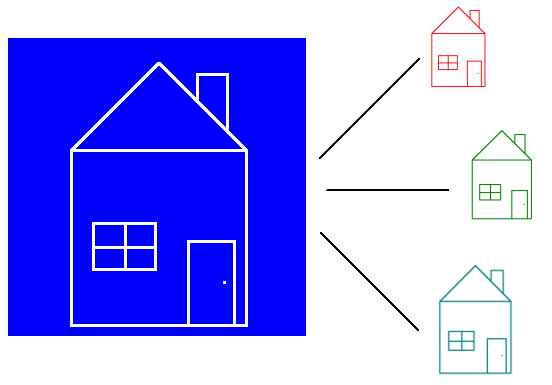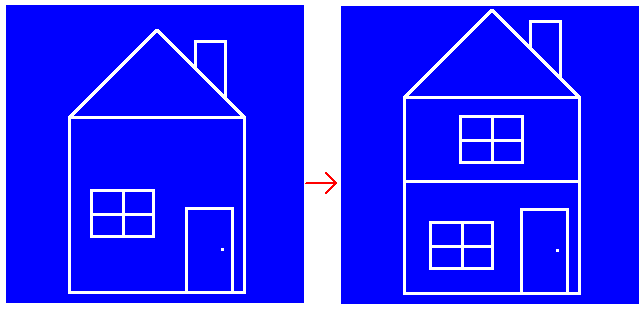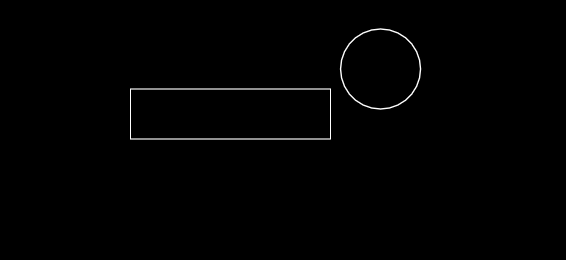Learn how to program games with the LÖVE framework

# Chapter 11 - Classes

Classes are like blueprints. You can create multiple houses with one blueprint. Similarly, we can create multiple objects from one class.For classes we're going to use a library: classic.

Click on `classic.lua` and then on Raw, and copy the code.

Go to your text editor, create a new file called `classic.lua` and paste the code.

Now we have to require it.

``````function love.load()
Object = require "classic"
end``````

And now we're ready to make a class. Create a new file called `rectangle.lua`, and put in the following code:

``````--! file: rectangle.lua

-- Pass Object as first argument.
Rectangle = Object.extend(Object)

function Rectangle.new(self)
self.test = math.random(1, 1000)
end``````

Everything will be explained. But first put this code in your `main.lua`.

``````--! file: main.lua
function love.load()
Object = require "classic"
--Don't forget to load the file
require "rectangle"

r1 = Rectangle()
r2 = Rectangle()
print(r1.test, r2.test)
end``````

When you run the game, you´ll see that 2 random numbers are printed.

So let's go through this code step by step. First we create a new class with `Rectangle = Object.extend(Object)`. This makes `Rectangle` become a class. This will be our blueprint. As opposed to properties, classes are usually written with an uppercase character (so this would be UpperCamelCase or PascalCase).

In `main.lua` we say `r1 = Rectangle()`. Even though `Rectangle` is a table, we can still call it as if it's a function. How that works is for another chapter. But by calling `Rectangle()` it creates a new instance. What that means is that it takes our blueprint and creates a new object with all the class its features. Every new instance is unique.

To prove that `r1` is unique, we create another instance called `r2`. Both have the property `test`, but they have different values.

When we call `Rectangle()`, it executes `Rectangle.new`. This is what we call the constructor.

The parameter `self`, is the instance we're modifying. If we were to type `Rectangle.test = math.random(0, 1000)`, we would be giving the property to the blueprint, and not to an instance made with the blueprint.

Let's make some changes to our class:

``````--! file: rectangle.lua
Rectangle = Object.extend(Object)

function Rectangle.new(self)
self.x = 100
self.y = 100
self.width = 200
self.height = 150
self.speed = 100
end

function Rectangle.update(self, dt)
self.x = self.x + self.speed * dt
end

function Rectangle.draw(self)
love.graphics.rectangle("line", self.x, self.y, self.width, self.height)
end``````

It's like the moving rectangle object we made in chapter 8. Except this time we put the code of the movement and drawing part in the object. Now we only need to call update and draw in `main.lua`.

``````--! file: main.lua

function love.load()
Object = require "classic"
require "rectangle"
r1 = Rectangle()
r2 = Rectangle()
end

function love.update(dt)
r1.update(r1, dt)
end

function love.draw()
r1.draw(r1)
end``````

When you run the game you'll see a moving rectangle.

So we created a class called `Rectangle`. We create an instance of that class called `r1`. So now `r1` has the functions `update` and `draw`. We call these functions, and as first argument we pass the instance itself, `r1`. This is what `self` becomes in the functions.

It's kind of annoying though, how we have to pass `r1` every time we call one of its functions. Luckily, Lua has a shorthand for this. When we use a colon (:), the function-call will automatically pass the object left of the colon as first argument.

``````--! file: main.lua
function love.update(dt)
--Lua turns this into: r1.update(r1, dt)
r1:update(dt)
end

function love.draw()
--Lua turns this into: r1.draw(r1)
r1:draw()
end``````

And we can do this with the functions as well.

``````--! file: rectangle.lua

--Lua turns this into: Object.extend(Object)
Rectangle = Object:extend()

--Lua turns this into: Rectangle.new(self)
function Rectangle:new()
self.x = 100
self.y = 100
self.width = 200
self.height = 150
self.speed = 100
end

--Lua turns this into: Rectangle.update(self, dt)
function Rectangle:update(dt)
self.x = self.x + self.speed * dt
end

--Lua turns this into: Rectangle.draw(self)
function Rectangle:draw()
love.graphics.rectangle("line", self.x, self.y, self.width, self.height)
end``````

We call this Syntactic sugar

Let's add some parameters to `Rectangle:new()`

``````--! file: rectangle.lua
function Rectangle:new(x, y, width, height)
self.x = x
self.y = y
self.width = width
self.height = height
self.speed = 100
end``````

With this we can give `r1` and `r2` each their own position and size.

``````--! file: main.lua

function love.load()
Object = require "classic"
require "rectangle"
r1 = Rectangle(100, 100, 200, 50)
r2 = Rectangle(350, 80, 25, 140)
end

function love.update(dt)
r1:update(dt)
r2:update(dt)
end

function love.draw()
r1:draw()
r2:draw()
end``````

So now we have 2 moving rectangles. This is what makes classes so great. `r1` and `r2` are the same, yet they are unique.Another thing that makes classes great is inheritance.

## Inheritance

With inheritance, we can extend our class. In other words, we make a copy of our blueprint, and add features to it, without editing the original blueprint.Let's say you have a game with monsters. Every monster has their own attack, they move differently. But they can also get damage, and are able to die. These overlapping features should be put in what we call a superclass or base class. They provide the features that all monsters have. And then each monster's class can extend this base class and add their own features to it.

Let's create another moving shape, a circle. What will our moving rectangle and circle have in common? Well they will both move. So let's make a base class for both of our shapes.

Create a new file called `shape.lua`, and put in the following code:

``````--! file: shape.lua
Shape = Object:extend()

function Shape:new(x, y)
self.x = x
self.y = y
self.speed = 100
end

function Shape:update(dt)
self.x = self.x + self.speed * dt
end``````

Our base class `Shape` now handles the movement. I should point out that base class is just a term. "X is a base class of Y". A base class is still the same like any other class. It's just different in how we use it.

Anyway, now that we have a base class that handles our movement, we can make `Rectangle` an extension of `Shape`, and remove its updater. Make sure to require `shape` before using it.

``````--! file: main.lua

function love.load()
Object = require "classic"
require "shape"
require "rectangle"
r1 = Rectangle()
r2 = Rectangle()
end``````
``````--! file: rectangle.lua
Rectangle = Shape:extend()

function Rectangle:new(x, y, width, height)
Rectangle.super.new(self, x, y)
self.width = width
self.height = height
end

function Rectangle:draw()
love.graphics.rectangle("line", self.x, self.y, self.width, self.height)
end``````

With `Rectangle = Shape:extend()` we made `Rectangle` an extension of `Shape`.

`Shape` has its own function called `:new()`. By creating `Rectangle:new()`, we override the original function. Meaning that when we call `Rectangle()`, it will not execute `Shape:new()` but instead `Rectangle:new()`.

But rectangle has the property `super`, which is the class `Rectangle` is extended from. With `Rectangle.super` we can get access to our base class's functions, and we use it to call `Shape:new()`.

We have to pass `self` as first argument ourselves, and can't let Lua handle it with a colon (:), because we're not calling the function as the instance.

Now we need to make a circle class. Create a new file called `circle.lua`, and put in the following code.

``````--! file: circle.lua
Circle = Shape:extend()

function Circle:new(x, y, radius)
Circle.super.new(self, x, y)
--A circle doesn't have a width or height. It has a radius.
self.radius = radius
end

function Circle:draw()
love.graphics.circle("line", self.x, self.y, self.radius)
end``````

So we make `Circle` an extension of `Shape`. We pass `x` and `y` to the `new()` function of `Shape` with `Circle.super.new(self, x, y)`.

We give our `Circle` class its own draw function. This is how you draw a circle. Circles don't have a width or height, they have a radius.

And now in `main.lua` load `shape.lua` and `circle.lua`, and change `r2` to a circle.

``````--! file: main.lua

function love.load()
Object = require "classic"
--Don't forget to load the file
require "shape"

require "rectangle"

--Don't forget to load the file
require "circle"

r1 = Rectangle(100, 100, 200, 50)

--We make r2 a Circle instead of a Rectangle
r2 = Circle(350, 80, 40)
end``````

Now when you run the game you'll see a moving rectangle and a moving circle.## One more time

Let's go through all this code one more time.

First we load the library classic with `require "classic"`. Loading this library returns a table, and we store this table inside `Object`. It has the very basics needed to simulate a class. Because Lua doesn't have classes, but by using `classic` we get a very nice imitation of a class.

Next we load `shape.lua`. In that file we create a new class called `Shape`. We will use this class as a base class for `Rectangle` and `Circle`. The 2 things that these classes have in common is that they have an `x` and `y` property, and that it moves horizontally. These similarities is what we put in `Shape`.

Next we create the `Rectangle` class. We make it an extension of our base class `Shape`. Inside the `:new()` function, the constructor, we call the constructor of our base class with `Rectangle.super.new(self, x, y)`. We pass `self` as first argument, so that `Shape` will use the instance of our blueprint, and not the blueprint itself. We give our rectangle a `width` and `height` property, and give it a draw function.

Next we repeat the above, except for a circle. So instead of a `width` and `height` we give it a `radius` property.

Now that we have our classes ready, we can start making instances of these classes. With `r1 = Rectangle(100, 100, 200, 50)` we create an instance of our class `Rectangle`. It is an object made out of our blueprint, and not the blueprint itself. Any changes we make to this instance will not affect the class. We update and draw this instance, and for that we use a colon (:). This is because we need to pass our instance as first argument, and the colon will make Lua do it for us.

And finally we do the same for `r2`, except we make it a `Circle`.

## Confused?

That was a lot of information for 1 chapter, and I can imagine if you're having a hard time understanding all of this. My advice: Keep following the tutorials. If you're new to programming it will take some time before you get all these new concepts, but eventually you'll get comfortable with them. I will keep adding explanations on older subjects while talking about the newer ones.

## Summary

Classes are like blueprints. We can create multiple objects out of 1 class. To simulate classes we use the library classic. You create a class with `ClassName = Object:extend()`. You create an instance of a class with `instanceName = ClassName()`. This will call the function `ClassName:new()`. This is called the constructor. Every function of a class should start with the parameter `self` so that when calling the function you can pass the instance as first argument. `instanceName.functionName(instanceName)`. We can use colons (:) to make Lua do this for us.

We can extend a class with `ExtensionName = ClassName:extend()`. This makes `ExtensionName` a copy of `ClassName` that we can add properties to without editing `ClassName`. If we give `ExtensionName` a function that `ClassName` already has, we can still call the original function with `ExtensionName.super.functionName(self)`.

Do you need help or do you see a mistake?
Leave a comment or edit this chapter.